Anzeige

# Testing of Hypothesis (1) (1).pptx

28. Mar 2023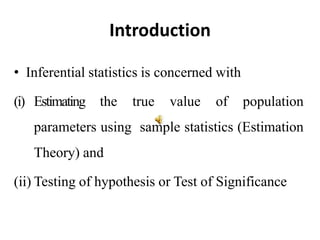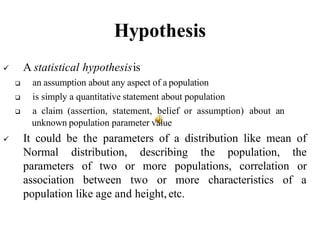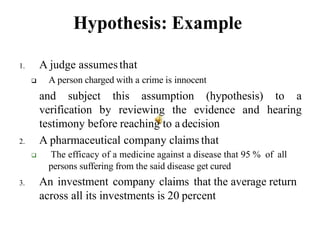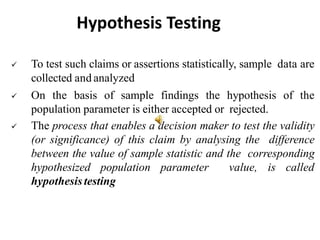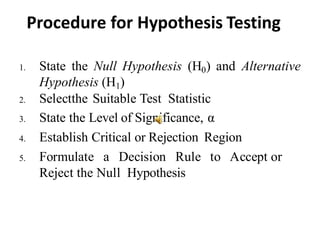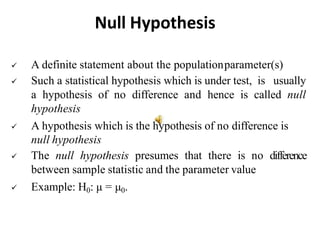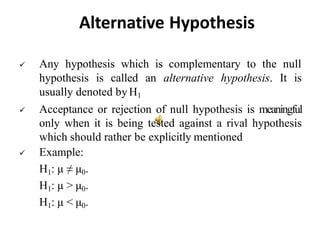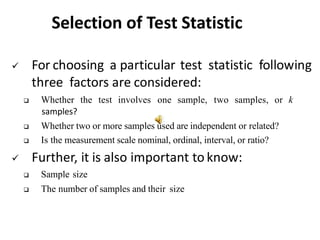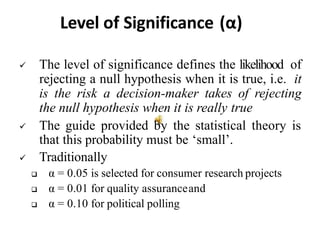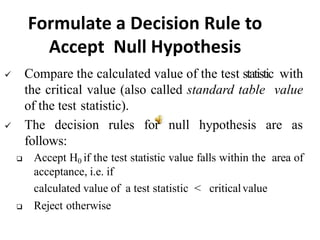1 von 12
Anzeige

### Testing of Hypothesis (1) (1).pptx

1. Testing Of Hypothesis Shri Vaishnav Institute of Management, Indore Department of Computer Science MCA I Year I Semester 2022-23 MCA-102 : Statistical Mathematics Unit-III By Dr. Bharti Agrawal Assistant Professor
2. Introduction • Inferential statistics is concerned with (i) Estimating the true value of population parameters using sample statistics (Estimation Theory) and (ii) Testing of hypothesis or Test of Significance
3. Hypothesis  A statistical hypothesisis  an assumption about any aspect of a population  is simply a quantitative statement about population  a claim (assertion, statement, belief or assumption) about an unknown population parameter value  It could be the parameters of a distribution like mean of Normal distribution, describing the population, the parameters of two or more populations, correlation or association between two or more characteristics of a population like age and height, etc.
4. Hypothesis: Example 1. A judge assumesthat  A person charged with a crime is innocent and subject this assumption (hypothesis) to a verification by reviewing the evidence and hearing testimony before reaching to a decision 2. A pharmaceutical company claims that  The efficacy of a medicine against a disease that 95 % of all persons suffering from the said disease get cured 3. An investment company claims that the average return across all its investments is 20 percent
5. Hypothesis Testing  To test such claims or assertions statistically, sample data are collected and analyzed  On the basis of sample findings the hypothesis of the population parameter is either accepted or rejected.  The process that enables a decision maker to test the validity (or significance) of this claim by analysing the difference between the value of sample statistic and the corresponding hypothesized population parameter value, is called hypothesis testing
6. Procedure for Hypothesis Testing 1. State the Null Hypothesis (H0) and Alternative Hypothesis (H1) 2. Selectthe Suitable Test Statistic 3. State the Level of Significance, α 4. Establish Critical or Rejection Region 5. Formulate a Decision Rule to Accept or Reject the Null Hypothesis
7. Null Hypothesis  A definite statement about the populationparameter(s)  Such a statistical hypothesis which is under test, is usually a hypothesis of no difference and hence is called null hypothesis  A hypothesis which is the hypothesis of no difference is null hypothesis  The null hypothesis presumes that there is no difference between sample statistic and the parameter value  Example: H0: μ = μ0.
8. Alternative Hypothesis  Any hypothesis which is complementary to the null hypothesis is called an alternative hypothesis. It is usually denoted by H1  Acceptance or rejection of null hypothesis is meaningful only when it is being tested against a rival hypothesis which should rather be explicitly mentioned  Example: H1: μ ≠ μ0. H1: μ > μ0. H1: μ < μ0.
9. Selection of Test Statistic  For choosing a particular test statistic following three factors are considered:  Whether the test involves one sample, two samples, or k samples?  Whether two or more samples used are independent or related?  Is the measurement scale nominal, ordinal, interval, or ratio?  Further, it is also important to know:  Sample size  The number of samples and their size
10. Level of Significance (α)  The level of significance defines the likelihood of rejecting a null hypothesis when it is true, i.e. it is the risk a decision-maker takes of rejecting the null hypothesis when it is really true  The guide provided by the statistical theory is that this probability must be ‘small’.  Traditionally  α = 0.05 is selected for consumer research projects  α = 0.01 for quality assuranceand  α = 0.10 for political polling
11. Critical Region or Rejection Region  The rejection region is the range of sample statistic values within which if values of the sample statistic falls (i.e. outside the limits of the acceptance region), then null hypothesis is rejected.  The value of the sample statistic that separates the regions of acceptance and rejection is called critical value.
12. Formulate a Decision Rule to Accept Null Hypothesis  Compare the calculated value of the test statistic with the critical value (also called standard table value of the test statistic).  The decision rules for null hypothesis are as follows:  Accept H0 if the test statistic value falls within the area of acceptance, i.e. if calculated value of a test statistic < criticalvalue  Reject otherwise
Anzeige